# ISEE Middle Level Quantitative : Distributive Property

## Example Questions

← Previous 1

### Example Question #1 : How To Find The Distributive Property

Which is the greater quantity?

(a)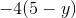(b)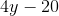(b) is greater

It is impossible to tell from the information given

(a) and (b) are equal

(a) is greater

(a) and (b) are equal

Explanation:

Apply the distributive and commutative properties to the expression in (a):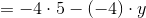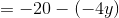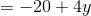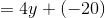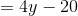The two expressions are equivalent.

### Example Question #2 : How To Find The Distributive Property

Which is the greater quantity?

(a)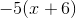(b)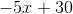(a) is greater

(b) is greater

(a) and (b) are equal

(b) is greater

Explanation:

Apply the distributive property to the expression in (a):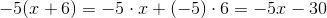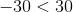, so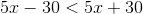regardless of.

Therefore,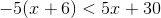### Example Question #1 : How To Find The Distributive Property

Which is the greater quantity?

(a)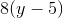(b)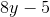(a) and (b) are equal

(a) is greater

(b) is greater

It is impossible to tell from the information given

(b) is greater

Explanation:

Apply the distributive property to the expression in (a):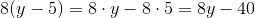Since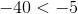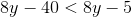, and therefore, regardless of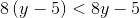### Example Question #1 : How To Find The Distributive Property

Which is the greater quantity?

(a)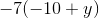(b)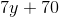It is impossible to tell from the information given

(b) is greater

(a) is greater

(a) and (b) are equal

It is impossible to tell from the information given

Explanation:

We show that there is at least one value ofthat makes the (a) greater and at least one that makes (b) greater:

Case 1: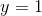(a)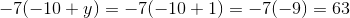(b)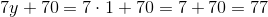(b) is greater here

Case 2: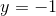(a)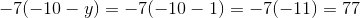(b)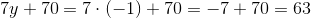(a) is greater here

### Example Question #5 : How To Find The Distributive Property

Which of the following is equivalent to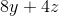?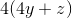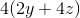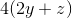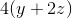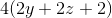Explanation:

We can best solve this by factoring 4 from both terms, and distributing it out: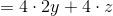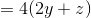### Example Question #6 : How To Find The Distributive Propertyandare positive integers.

Which of the following is greater?

(A)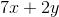(b)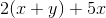(A) is greater

(A) and (B) are equal

It is impossible to determine which is greater from the information given

(B) is greater

(A) and (B) are equal

Explanation: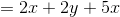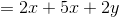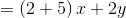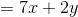(A) and (B) are equivalent variable expressions and are therefore equal regardless of the values ofand.

### Example Question #7 : How To Find The Distributive Property

Simplify the below: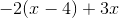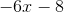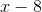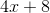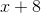Explanation:

In order to simiplify we must first distribute the -2 only to what is inside the ( ):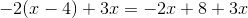Now, we must combine like terms: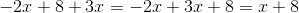This gives us the final answer:### Example Question #8 : How To Find The Distributive Property

Simplify the below: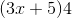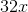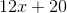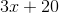This does not simplifyExplanation:

We must use the distributive property in this case to multiply the 4 by both the 3x and 5.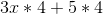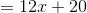### Example Question #9 : How To Find The Distributive Propertyand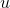are positive numbers. Which is the greater quantity?

(a)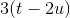(b)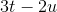It is impossible to determine which quantity is the greater from the information given

(a) and (b) are equal

(a) is the greater quantity

(b) is the greater quantity

(b) is the greater quantity

Explanation: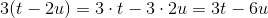Sinceis positive, and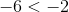, then, by the properties of inequality,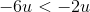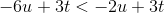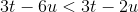and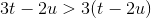.

### Example Question #10 : How To Find The Distributive Propertyis the additive inverse of. Which is the greater quantity?

(a)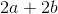(b)It is impossible to determine which is greater from the information given

(a) and (b) are equal

(a) is the greater quantity

(b) is the greater quantityis the additive inverse of, so, by definition,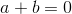.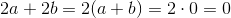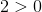.